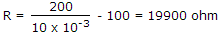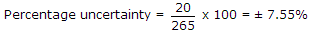Top

# Measurements and Instrumentation

1.

Assertion (A): Schering bridge is a low voltage bridge.

Reason (R): Schering bridge is very commonly used for measurement of capacitance.

Schering bridge is a high voltage bridge.

#### Enter details here

2.

Two numbers are 131 ± 5 and 629 ± 3. The sum of the numbers with range of doubt is

The numbers can be 131 + 5, 131 - 5, 629 - 3. Therefore, the sum can be 760 ± 8.

#### Enter details here

3.

Which of these has a magnetic brake?

Braking torque, in an energy meter, is provided by a magnet.

#### Enter details here

4.

A meter coil has a resistance of 100 Ω and a full scale deflection current of 10 mA. To convert it into a voltmeter of 0-200 V range, the series resistance should be.

#### Enter details here

5.

The power input and output in a device are 3650 W and 3385 W with each reading in doubt by ± 10 W. The percentage uncertainty in losses is.